# C++ 入门教程之六 -- Classes 实践/头文件/操作符复用

### 新建项目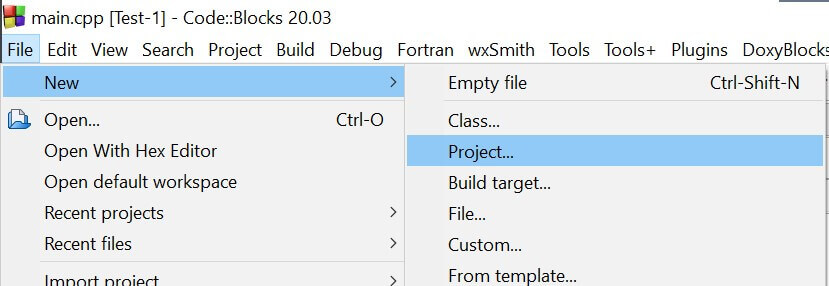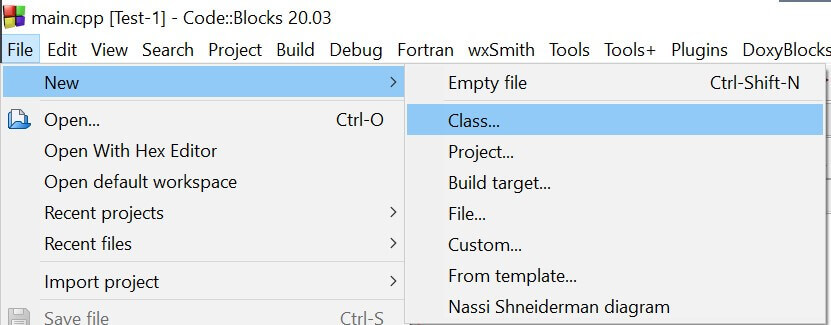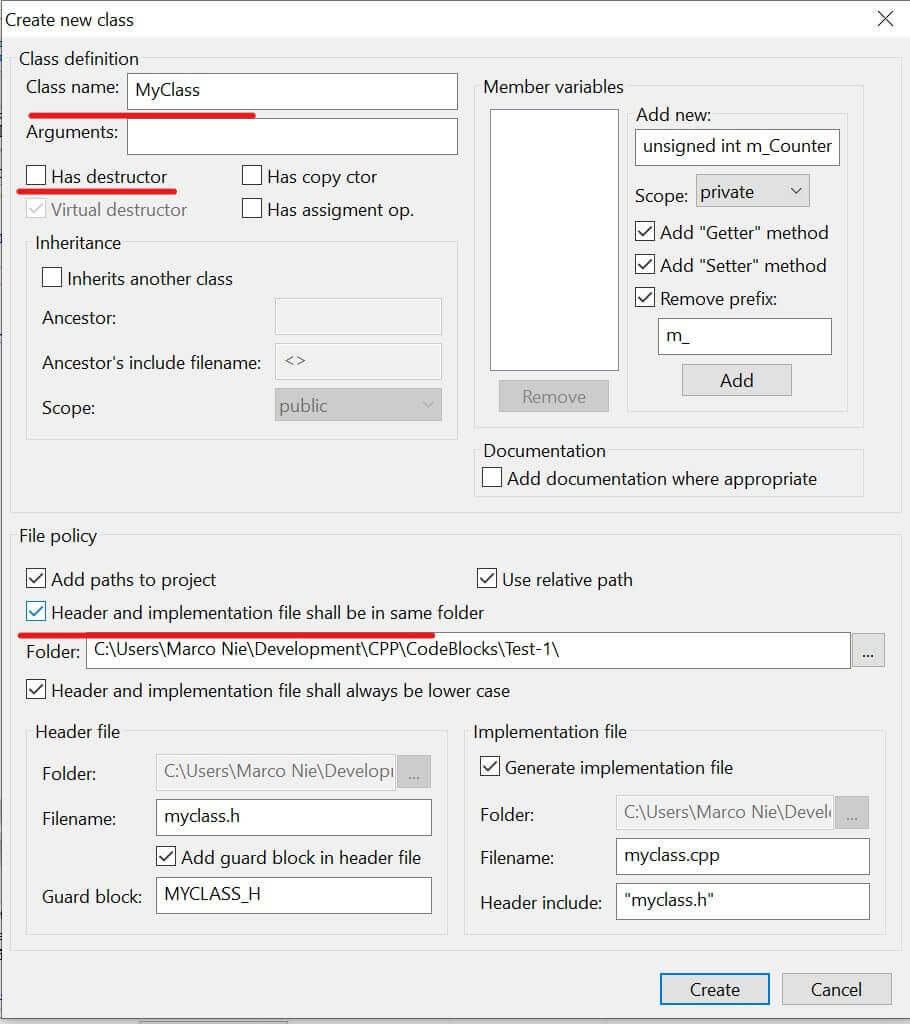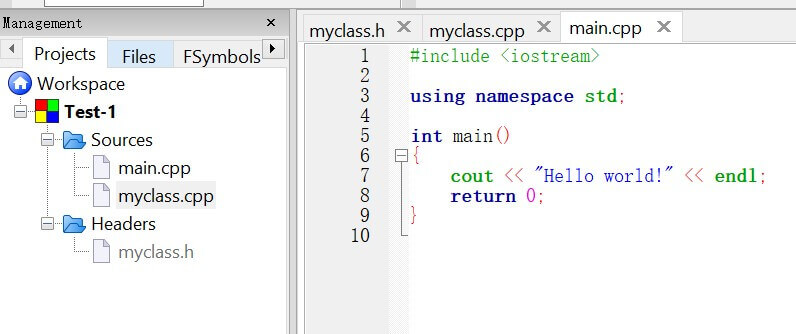• main.cpp 项目主文件
• MyClass.cpp class source 源文件

### 头文件和源文件

``````#ifndef MYCLASS_H
#define MYCLASS_H

class MyClass
{
public:
MyClass();

protected:

private:
};

#endif // MYCLASS_H``````

``````#include "myclass.h"

MyClass::MyClass()
{
//ctor
}``````

``````//定义一个元素为class内的一个att1类型：
MyClass1::att1 a;

//使用class的function：
MyClass2 obj;
obj.func();``````

``````#include <iostream>
#include "myclass.h"

using namespace std;

int main()
{
MyClass obj;
return 0;
}``````

### destructor 销毁器

``````class MyClass {
public:
~MyClass() {
// some code
}
};``````

``````class MyClass
{
public:
MyClass();
~MyClass();
};``````

``````#include "MyClass.h"
#include <iostream>
using namespace std;

MyClass::MyClass()
{
cout<<"Constructor"<<endl;
}

MyClass::~MyClass()
{
cout<<"Destructor"<<endl;
}``````

``````Constructor
Destructor``````

### 选择符

``````MyClass obj;
MyClass *ptr = &obj;
ptr->myPrint();``````

object 元素访问基本原则：

• 如果直接面对 object，使用`.` 来访问 object 的元素，如：`obj.myPrint()`
• 如果使用面对指针指向的 object，使用选择符`->` 来访问 object 的元素，如：`ptr->myPrint()`

### constants 常数

``````const int a=2;
``````

``````const MyClass obj;
``````

object 内所有的 variable 变量必须在初始化时赋值，一般在 constructor 构造器内完成，实例化的时候直接给其传递数据。如果没有提供构造器完成参数初始化赋值，会引起编译器报错。

``````class MyClass
{
public:
void myPrint() const;
};``````

``````#include "MyClass.h"
#include <iostream>
using namespace std;

void MyClass::myPrint() const {
cout <<"Hello"<<endl;
}``````

``````int main() {
const MyClass obj;
obj.myPrint();
}
// Outputs "Hello"``````

### 元素初始化器

const 类型的元素数据不能够被改变，且必须在创建时赋值。c++ 提供了一个语法结构来给 class 内元素进行初始化叫做：constructor initializer list 构造器初始化列表。

``````class MyClass {
public:
MyClass(int a, int b) {
regVar = a;
constVar = b;
}
private:
int regVar;
const int constVar;
};``````

const 类型元素在申明后不能再对其修改。

``````class MyClass {
public:
MyClass(int a, int b)
: regVar(a), constVar(b)
{
}
private:
int regVar;
const int constVar;
};``````

MyClass.h:

``````#ifndef MYCLASS_H
#define MYCLASS_H

class MyClass
{
public:
MyClass(int a, int b);
~MyClass();

protected:

private:
int regVar;
const int constVar;
};

#endif // MYCLASS_H``````

MyClass.cpp:

``````#include "myclass.h"
#include <iostream>

using namespace std;

MyClass::MyClass(int a, int b)
: regVar(a), constVar(b)
{
cout << regVar << endl;
cout << constVar << endl;
}

MyClass::~MyClass()
{
cout << "destructor" << endl;
}``````

main.cpp：

``````#include <iostream>
#include "myclass.h"

using namespace std;

int main()
{
MyClass obj(42, 33);

return 0;
}``````

``````42
33
destructor``````

### 结构化

birthday.h:

``````#ifndef BIRTHDAY_H
#define BIRTHDAY_H

class Birthday
{
public:
Birthday(int m, int d, int y);
void printDate();

protected:

private:
int month;
int day;
int year;
};

#endif // BIRTHDAY_H``````

birthday.cpp: 注意将 function 返回类型放在范围解析符前

``````#include "birthday.h"
#include <iostream>

using namespace std;

Birthday::Birthday(int m, int d, int y)
: month(m), day(d), year(y)
{
}

void Birthday::printDate()
{
cout << month << "/" << day << "/" << year << endl;
}``````

persion.h:

``````#ifndef PERSON_H
#define PERSON_H
#include "birthday.h"
#include <string>

using namespace std;

class Person
{
public:
Person(string n, Birthday b);
void printInfo();

protected:

private:
string name;
Birthday bd;
};

#endif // PERSON_H``````

persion.cpp:

``````#include "person.h"
#include <iostream>

using namespace std;

Person::Person(string n, Birthday b)
: name(n), bd(b)
{
}

void Person::printInfo()
{
cout << name << endl;
bd.printDate();
}``````

Person 包含 name 和 Birthday 两个元素，且在构造器内对其初始化。

``````#include "person.h"
#include "birthday.h"

using namespace std;

int main()
{
Birthday bd(11, 25, 1989);
Person p("Marco", bd);
p.printInfo();

return 0;
}``````

### friend 关键词

``````#ifndef MYCLASS_H
#define MYCLASS_H

class MyClass
{
public:
MyClass(int a, int b);
~MyClass();

protected:

private:
int regVar;
const int constVar;

};

#endif // MYCLASS_H
};``````

``````#include "myclass.h"
#include <iostream>

using namespace std;

{
cout << obj->constVar << endl;
}

int main()
{
MyClass a(42, 33);
MyClass *b = &a;

return 0;
}``````

``````33
``````

friend function 不属于任何 class。

### this 关键词

``````class MyClass {
public:
MyClass(int a)
: var(a)
{ }
void printInfo() {
cout << var << endl;
cout << this->var << endl;
cout << (*this).var << endl;
}
private:
int var;
};``````

`printInfo()` 中的三条指令会得到相同的结果。this 是一个指针，所以使用 `->` 来读取对应地址的数据，也可以使用 `*this` 数据查询符来表示此地址下的数据。可以参考教程：C++ 入门教程 -- 数据类型，数组及指针

### 操作符复用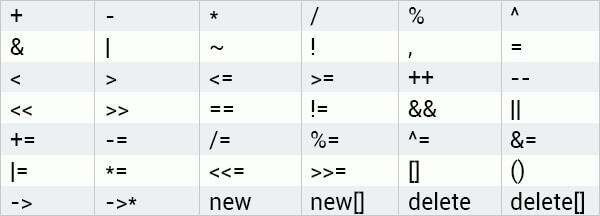``````class MyClass {
public:
int var;
MyClass(int a)
: var(a)
{ }
};``````

``````class MyClass {
public:
int var;
MyClass(int a)
: var(a) { }

MyClass operator+(MyClass &obj) {
MyClass res;
res.var = this->var+obj.var;
return res;
}
};``````

``````int main() {
MyClass obj1(12), obj2(55);
MyClass res = obj1 + obj2;

cout << res.var;
}

//Outputs 67``````